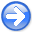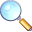Madagascar tutorialNext: Exercise: convert command-line arguments Up: Maurice: Tutorial Previous: Warm-up

# The RSF file format

model
Figure 1.
Stratigraphic layers overlayed on amodel.Next we will try to generate a 2D synthetic model from ASCII files.

1. Change directory to the project directory
```bash\$ cd asc2rsf
```
2. Run
```bash\$ scons view
```
will generate a synthetic model that looks like Figure 1 on the screen.
3. To understand how the model is created, examine the SConstruct script. ASCII files inp*.asc are created using a python loop.
4. The ASCII files are converted into the RSF file format and then interpolated using 1-D cubic spline interpolation:
```bash\$ sfdd form=native <inp0.asc | \
sfspline o1=0 d1=0.05 n1=201 fp=0,0 >lay1.rsf
```
5. The layer files are gathered into a single RSF file by arranging them along the second dimension
```bash\$ sfcat axis=2 < lay1.rsf lay2.rsf lay3.rsf lay4.rsf > lays.rsf
```
6. The layered gradient () velocity model is created independently using sfmath, a very useful command in Madagascar that performs common mathematical operations on RSF files.
7. The final figure is created by overlaying the layers onto the velocity model using the Overlay plotting option.Madagascar tutorialNext: Exercise: convert command-line arguments Up: Maurice: Tutorial Previous: Warm-up

2015-01-08